# GRE Algebra | Graph of Functions

• Last Updated : 05 Jan, 2021

Graphs hold a very important place in the world of Algebra and are crucially components of GRE preparation. Graph functions are simple and must be understood carefully to successfully get correct answers in GRE. They are not difficult and once understood, will help in fetching marks easily.

Below are examples of some well known graphs of functions:

1. Linear fxn: Straight line graph where m is the slope and c represents the intercept in

`y=mx+c`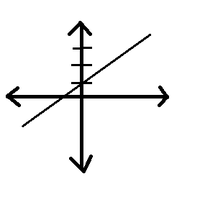Graph for Liner fxn (y=mx+c)

2. Square fxn: Shape of the graph is parabola.

`y=x^2`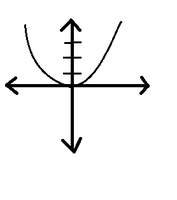Graph for Square fxn (x^2)

3. Square Root fxn: Such graphs always lie in first quadrant.

`y=x^1/2`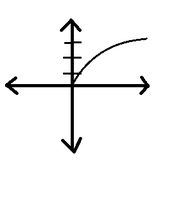Graph for Square Root fxn (x^1/2)

4. Cube fxn

`y=x^3`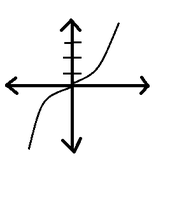Graph for cube fxn (x^3)

5. Exponential fxn

`y=e^x`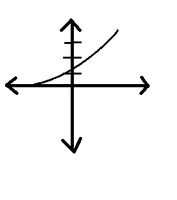Graph for Exponential (e^x)

6. Logarithmic fxn

`y=ln(x)`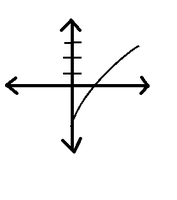Graph for logarithmic x

7. Modulo fxn

`y=|x|`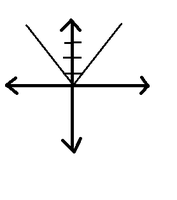Graph for |x|

8. Sine fxn

`y=sin(x)`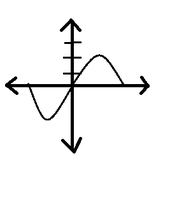Graph for sin x

9. Cosine fxn

`y=cos(x)`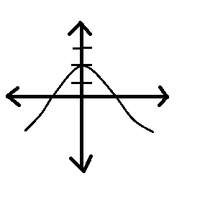Graph for  cos x

10. Tangent fxn

`y=tan(x)`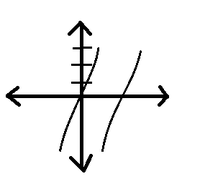Graph for tan x

My Personal Notes arrow_drop_up